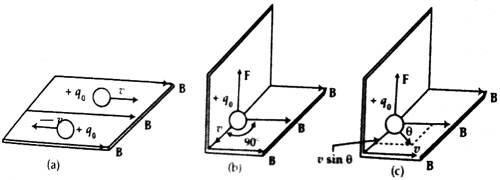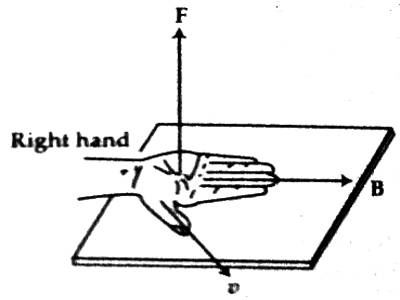# Explanation of Moving Charge

Explanation of Moving Charge

We know that current is produced due to the flow of charges. Again, a magnetic field is produced when current flows through a conductor. So, it can be said that moving charge creates a magnetic field.

Magnitude and direction of the Magnetic force on a charge moving in a magnetic field.

Explanation: In figure (a), a charge is moving with velocity v parallel to the magnetic field. Although the charge is mobile, no magnetic force will act on it, i.e., the charge will not experience any force. This is because that the velocity is zero perpendicular to the magnetic field: so the second condition mentioned above is not fulfilled. In figure (b) and (c) a charge is in motion making angles 90° and θ respectively with the magnetic field. In both cases, the charge will experience the magnetic force. But the force will be maximum when the angle between the magnetic field and the velocity is 90°. In that case, the velocity is maximum along the normal to the magnetic field.The direction of the magnetic force will be perpendicular to both velocity v and magnetic field B. That means F is perpendicular to the plane containing v and B. In order to determine F right-hand rule-I (RHR-1) is to be applied [Figure – 2].

Right-hand rule-1: When the right hand is stretched, if the fingers indicate the magnetic field and the thumb indicate the velocity of the charge, then outward normal drawn on the palm will indicate the direction of force for the positive charge. In case of a negative charge, it will be reversed i.e., the direction of force will be inward normal.By analyzing the motion of charged particle in a uniform magnetic field it is known that,

(i) the magnitude of magnetic force F is proportional to the charge q, speed v and the magnitude of the magnetic field,

(ii) the magnetic force is proportional to the sine of the angle between the direction of velocity v and the magnetic field B.